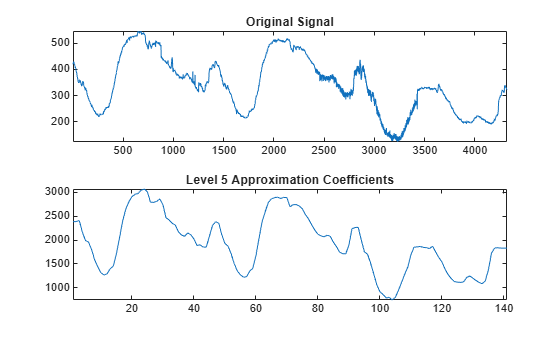# appcoef

1-D approximation coefficients

## Syntax

``A = appcoef(c,l,wname)``
``A = appcoef(c,l,LoR,HiR)``
``A = appcoef(___,N)``
``A = appcoef(___,Mode=extmode)``

## Description

````A = appcoef(c,l,wname)` returns the approximation coefficients at the coarsest scale using the wavelet decomposition structure [`c`,`l`] of a 1-D signal and the wavelet specified by `wname`. For more information, see `wavedec`.```
````A = appcoef(c,l,LoR,HiR)` uses the lowpass reconstruction filter `LoR` and highpass reconstruction filter `HiR`.```

example

````A = appcoef(___,N)` returns the approximation coefficients at level `N`. If [`c`,`l`] is the `M`-level wavelet decomposition structure of a 1-D signal, then `0 ≤ N ≤ M = length(l)-2`.```
````A = appcoef(___,Mode=extmode)` uses the specified discrete wavelet transform (DWT) extension mode `extmode`. This syntax can be used with either of the previous syntaxes.```

## Examples

collapse all

Load the signal consisting of electricity usage data.

`load leleccum`

Obtain the DWT down to level 5 using the `sym4` wavelet.

`[c,l] = wavedec(leleccum,5,"sym4");`

Extract the approximation coefficients at the coarsest scale. Plot the original signal and the approximation coefficients.

```lev = 5; a3 = appcoef(c,l,"sym4",lev); tiledlayout(2,1) nexttile plot(leleccum) axis tight title("Original Signal") nexttile plot(a3) axis tight title("Level "+num2str(lev)+" Approximation Coefficients")```## Input Arguments

collapse all

Wavelet decomposition vector of a 1-D signal, specified as a vector. For more information, see `wavedec`.

Example: `[c,l] = wavedec(randn(1,256),4,"coif1")` returns the wavelet decomposition of a vector down to level 4.

Data Types: `single` | `double`
Complex Number Support: Yes

Bookkeeping vector of the wavelet decomposition of a 1-D signal, specified as a vector of positive integers. The bookkeeping vector is used to parse the coefficients in the wavelet decomposition vector `c` by level. For more information, see `wavedec`.

Data Types: `single` | `double`

Wavelet used to generate the wavelet decomposition [`c`,`l`], specified as a character vector or string scalar. The wavelet is from one of the following wavelet families: Best-localized Daubechies, Beylkin, Coiflets, Daubechies, Fejér-Korovkin, Haar, Han linear-phase moments, Morris minimum-bandwidth, Symlets, Vaidyanathan, Discrete Meyer, Biorthogonal, and Reverse Biorthogonal. See `wavemngr` for the wavelets available in each family.

Example: `"db4"`

Wavelet reconstruction filters, specified as a pair of even-length real-valued vectors. `LoR` is the lowpass reconstruction filter, and `HiR` is the highpass reconstruction filter. The lengths of `LoR` and `HiR` must be equal. For perfect reconstruction, `LoR` and `HiR` must be the reconstruction filters associated with the same wavelet used to obtain the wavelet decomposition `c` and `l`. For more information, see `wfilters`

Data Types: `single` | `double`

Approximation coefficients level, specified as a positive integer. If [`c`,`l`] is the `M`-level wavelet decomposition structure of a 1-D signal, then `0 ≤ N ≤ M`.

Since R2023b

Extension mode to use for the inverse DWT, specified as:

`mode`

DWT Extension Mode

`"zpd"`

Zero extension

`"sp0"`

Smooth extension of order 0

`"spd"` (or``` "sp1"```)

Smooth extension of order 1

`"sym"` or `"symh"`

Symmetric extension (half point): boundary value symmetric replication

`"symw"`

Symmetric extension (whole point): boundary value symmetric replication

`"asym"` or `"asymh"`

Antisymmetric extension (half point): boundary value antisymmetric replication

`"asymw"`

Antisymmetric extension (whole point): boundary value antisymmetric replication

`"ppd"`, `"per"`

Periodized extension

If the signal length is odd and `mode` is `"per"`, an extra sample equal to the last value is added to the right and the extension is performed in `"ppd"` mode. If the signal length is even, `"per"` is equivalent to `"ppd"`.

The global variable managed by `dwtmode` specifies the default extension mode. For perfect reconstruction, use the same extension mode that was used to obtain `c` and `l` from `wavedec`.

## Output Arguments

collapse all

Approximation coefficients at level `N`, returned as a vector.

## Algorithms

The input vectors `c` and `l` contain all the information about the signal decomposition.

Let `NMAX = length(l)-2`; then ```c = [A(NMAX) D(NMAX) ... D(1)]``` where `A` and the `D` are vectors. If `N = NMAX`, then a simple extraction is done; otherwise, `appcoef` computes iteratively the approximation coefficients using the inverse wavelet transform.

## Version History

Introduced before R2006a

expand all